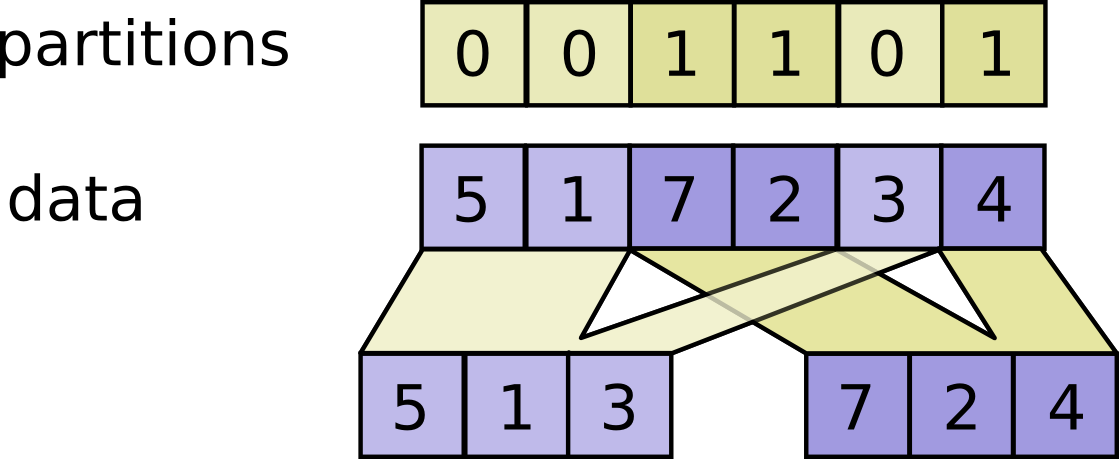# DynamicPartition

public final class DynamicPartition

Partitions `data` into `num_partitions` tensors using indices from `partitions`.

For each index tuple `js` of size `partitions.ndim`, the slice `data[js, ...]` becomes part of `outputs[partitions[js]]`. The slices with `partitions[js] = i` are placed in `outputs[i]` in lexicographic order of `js`, and the first dimension of `outputs[i]` is the number of entries in `partitions` equal to `i`. In detail,

``````outputs[i].shape = [sum(partitions == i)] + data.shape[partitions.ndim:]

outputs[i] = pack([data[js, ...] for js if partitions[js] == i])
``````

For example:

``````# Scalar partitions.
partitions = 1
num_partitions = 2
data = [10, 20]
outputs = []  # Empty with shape [0, 2]
outputs = [[10, 20]]

# Vector partitions.
partitions = [0, 0, 1, 1, 0]
num_partitions = 2
data = [10, 20, 30, 40, 50]
outputs = [10, 20, 50]
outputs = [30, 40]
``````
See `dynamic_stitch` for an example on how to merge partitions back.Raises: * `InvalidArgumentError` in following cases: - If partitions is not in range `[0, num_partiions)` - If `partitions.shape` does not match prefix of `data.shape` argument.

### Public Methods

 static DynamicPartition create(Scope scope, Operand data, Operand partitions, Long numPartitions) Factory method to create a class wrapping a new DynamicPartition operation. Iterator> iterator() List> outputs()

## Public Methods

#### public static DynamicPartition<T> create(Scope scope, Operand<T> data, Operand<Integer> partitions, Long numPartitions)

Factory method to create a class wrapping a new DynamicPartition operation.

##### Parameters
scope current scope Any shape. Indices in the range `[0, num_partitions)`. The number of partitions to output.
##### Returns
• a new instance of DynamicPartition

#### public List<Output<T>> outputs()

[{ "type": "thumb-down", "id": "missingTheInformationINeed", "label":"Missing the information I need" },{ "type": "thumb-down", "id": "tooComplicatedTooManySteps", "label":"Too complicated / too many steps" },{ "type": "thumb-down", "id": "outOfDate", "label":"Out of date" },{ "type": "thumb-down", "id": "samplesCodeIssue", "label":"Samples / code issue" },{ "type": "thumb-down", "id": "otherDown", "label":"Other" }]
[{ "type": "thumb-up", "id": "easyToUnderstand", "label":"Easy to understand" },{ "type": "thumb-up", "id": "solvedMyProblem", "label":"Solved my problem" },{ "type": "thumb-up", "id": "otherUp", "label":"Other" }]